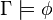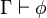# User:IssaRice/Computability and logic/Gödel's completeness theorem

1. (for all sets of sentences$\Gamma$ and all sentences$\phi$?) If$\Gamma \models \phi$, then$\Gamma \vdash \phi$